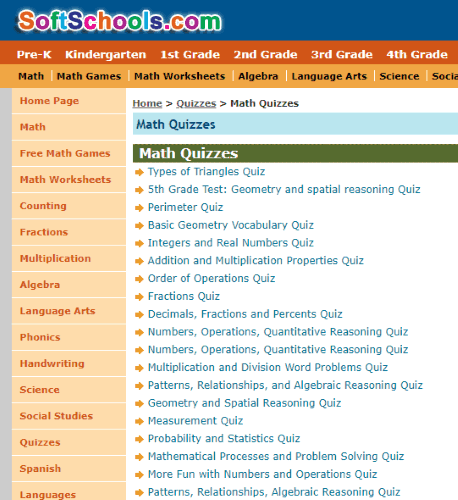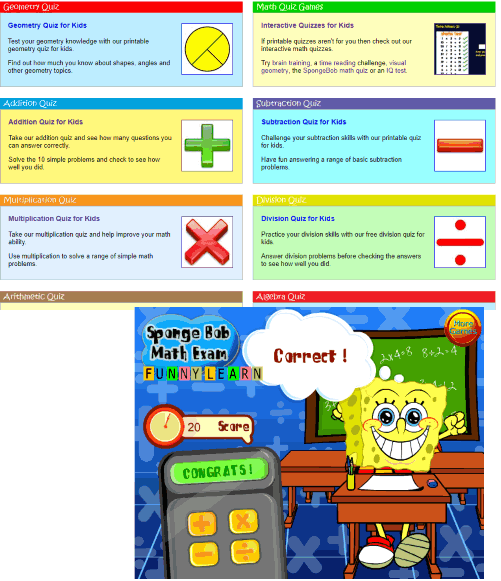Editor Ratings:
User Ratings:
[Total: 3 Average: 3.3]

Here are 5 Math quiz for kids free websites. These websites provide different types of random Math questions for kindergarten kids, grade A, grade B, grade C kids, etc. From addition to multiplication, subtraction to division, you can select the type of quiz, start the quiz and let your kid(s) complete that quiz by providing the answers. At the end of the quiz, the result is presented to see if the kid passed it or missed it. Some of the Math quiz for kids’ websites also let you take print of quiz result without any watermark.

These websites help your kids learn and practice Math in a fun way. It will also sharpen their brain and help to increase their interest in Math. You will need to enable Adobe Flash to begin the quiz for some of these websites.

So, let’s check Math quiz for kids provided by these free websites.

## Math4ChildrenMath4Children is one of the best websites for Math quizzes for kids. This website provides quizzes for pre-kindergarten kids, kindergarten kids, first grade to eighth grade Math quizzes, etc. For each age group, it brings different types of quizzes. For example, for the first grade Math quiz, there are counting, addition, subtraction, counting, fraction, and other quizzes available to choose from.

Select a quiz and then start giving the answers. Here, the good thing is the quiz is time-based. So, try to complete the quiz before time. Once the quiz is completed, it shows whether you passed it or not. You can also see the total number of questions, full score, passing rate, passing score, your score, and the total time elapsed to complete the quiz.

The feature to take print of quiz result without watermark is also provided. So, the website is pretty good and I can easily recommend it to you.

## SoftSchools.comSoftSchools.com is also a handy website to find and play Math quizzes for kids as well as elder students. There are around 100+ quizzes. You can start with triangles quiz, order of operations quiz, 3rd Grade probability quiz, numbers, decimals, 4th Grade word problem quiz, brain teaser quiz or any other quizzes available on the list.

For the selected quiz, there will be some random problems or questions which you need to answer. Some quizzes come with multi-answer questions so it becomes a bit easier to give the correct answer. When the quiz is completed, you can see the result which shows your total answers (including right and wrong answers), all the correct answers, and your result (pass or fail). The feature to save result is also present but for that, you need to create a free account.

Apart from tons of Math quizzes, this website also brings different Math games for kindergarten kids, pre-kindergarten kids, 1st grade to 5th grade, middle school, and more.

## ABCyaABCya is a very interesting website where kids can play a quiz for addition, division, subtraction, and multiplication. The best part is you can select the difficulty level before beginning the quiz. You can also type your name and begin the quiz. Based on the difficulty level and selected Math operation, 10 random questions are given to you. Solve the quiz as early as possible.

Finally, you can see the quiz result. The good thing is it shows your answers and correct answers side by side. Your quiz score, best score, and the total number of quizzes taken by you is also visible.

It also lets you take the print of quiz result where your name, quiz score, correct answers, and your answers are visible. The print won’t contain any watermark. So, the website is pretty helpful to play Math quiz for kids.

## KidzoneKidzone website provides basic facts Math quizzes. There are addition, multiplication, and subtraction quizzes available to play. The subtraction and addition problems are up to 18-18 and 18+18. And Multiplication problems are up to 10*10. The website doesn’t provide division quiz, which is a bit strange. Still, the website is handy for these three basic Math operations. For each type of quiz, you can select the total number of problems like 5, 35, 20, 10, 50, 100, etc.

Select an operation, number of problems and start the quiz. A separate window is opened to solve the quiz. Once all the answers are given, you can submit the answers and see the result. In the result, you will see how many problems were answered correctly along with the total time taken to solve the quiz. Finally, you can also take the print of the result. This way, you can begin with another quiz. Each time you start a quiz, random problems are generated. It doesn’t provide repetitive problems which is a good thing.

## Kids Math Games OnlineKids Math Games Online (Homepage) brings different types of quizzes. There is SpongeBob Math Quiz which brings random Math questions (addition, subtraction, multiplication, and division), Brain Training Math Exercises where some simple sums are provided, and Time Test for Kids where you can find the difference between start and end time.

It also comes with free IQ test and printable Math quizzes which includes basic Algebra quiz, Multiplication, Geometry quiz for kids, and more. If you are not interested in printable Math quizzes, then simply use Brain Training Math Exercises and SpongeBob Math Quiz section, and other sections to start playing quiz online.

We’ve also covered some free online Math games for you.

### The Conclusion:

These are some best picked websites to play Math quiz for kids. The interface to play Math quiz is user-friendly in all of these websites. While some websites provide basic Math operation quizzes, others are helpful to play simple to advanced Math quizzes. Among all these interesting websites, I find “Math4Children” pretty helpful than others.

 Editor Ratings: User Ratings:[Total: 3 Average: 3.3]